# Assignments

The data for this assignment are listed in the Excel file Quadratic fuel model.
Car designers have been experimenting with ways to improve gas mileage for many years. An important element in this research is the way in which a car’s speed affects how quickly fuel is burned. Competitions with the objective of driving the farthest on the smallest amount of gas have determined that low speeds and high speeds are inefficient. Designers would like to know which speed burns gas most efficiently. As an experiment, 50 identical cars are driven at different speeds and the gas mileage measured. They use the following model which may describe the relation between gas mileage and speed:

Mileage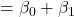speedspeed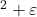a. Explain why the designers assumed that this model may be correct. Also, predict the sign of the coefficient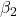without doing any computer computations.
b. Perform a regression analysis using your model and give the regression equation.
c. How well does it fit? Consider the coefficient of determination and the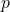-value of the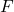-test.
d. Test the coefficients. What may you conclude from this?
e. If you accept this model, which speed would optimize the mileage and why?

Solution

0